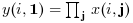Scilab Home page | Wiki | Bug tracker | Forge | Mailing list archives | ATOMS | File exchange
Scilab 5.5.2
Change language to: Français - Português - 日本語 - Русский

See the recommended documentation of this function

# prod

product of array elements

### Calling Sequence

```y=prod(x)
y=prod(x,orientation)
y=prod(x,outtype)
y=prod(x,orientation,outtype)```

### Arguments

x

an array of reals, complex, booleans, polynomials or rational fractions.

orientation

it can be either

• a string with possible values `"*"`, `"r"`, `"c"` or `"m"`

• a number with positive integer value

outtype

a string with possible values `"native"` or `"double"`.

y

scalar or array

### Description

For an array `x`, `y=prod(x)` returns in the scalar `y` the product of all the elements of `x`.

`y=prod(x,orientation)` returns in `y` the product of `x` along the dimension given by `orientation`:

• if `orientation` is equal to 1 or "r" thenor• if `orientation` is equal to 2 or "c" then:or• if `orientation` is equal to n then• `y=prod(x,"*")` is equivalent to `y=prod(x)`

• `y=prod(x,"m")` is equivalent to `y=prod(x,orientation)` where `orientation` is the index of the first dimension of `x` that is greater than 1.

The `outtype` argument rules the way the product is done:

• For arrays of floats, of polynomials, of rational fractions, the evaluation is always done using floating points computations. The `"double"` or `"native"` options are equivalent.

• For arrays of integers,

if `outtype="native"` the evaluation is done using integer computations (modulo 2^b, where b is the number of bits used),

if `outtype="double"` the evaluation is done using floating point computations.

The default value is `outtype="native"`.

• For arrays of booleans,

if `outtype="native"` the evaluation is done using boolean computations (* is replaced by &),

if `outtype="double"` the evaluation is done using floating point computations (%t values are replaced by 1 and %f values by 0).

The default value is `outtype="double"`. This option is used for Matlab compatibility.

### Remark

This function applies, with identical rules to sparse matrices and hypermatrices.

### Examples

```A=[1,2;3,4];
prod(A)
prod(A,1)

I=uint8([2 95 103;254 9 0])
prod(I) //native evaluation
prod(I,"double")
prod(I,2,"double")

s=poly(0,"s");
P=[s,%i+s;s^2,1];
prod(P),
prod(P,2)

B=[%t %t %f %f];
prod(B) //evaluation in float
prod(B,"native") //similar to and(B)```